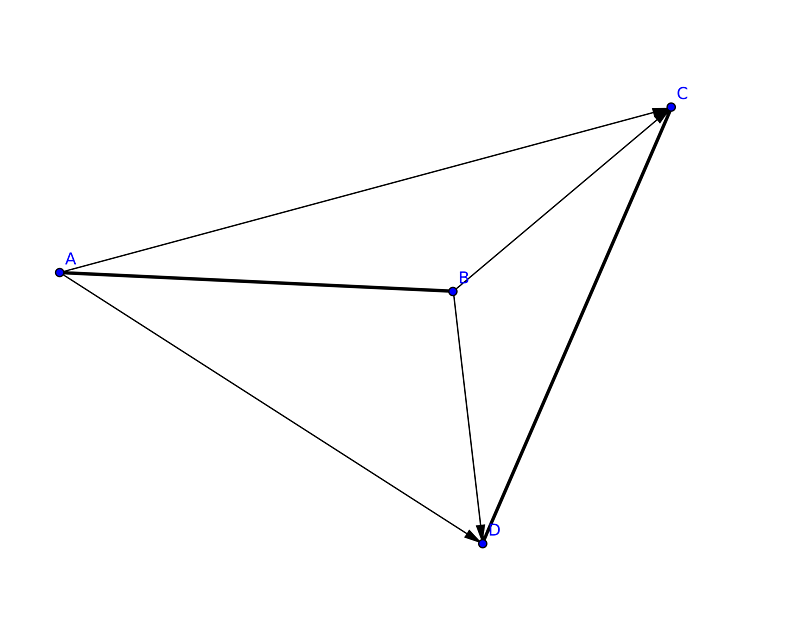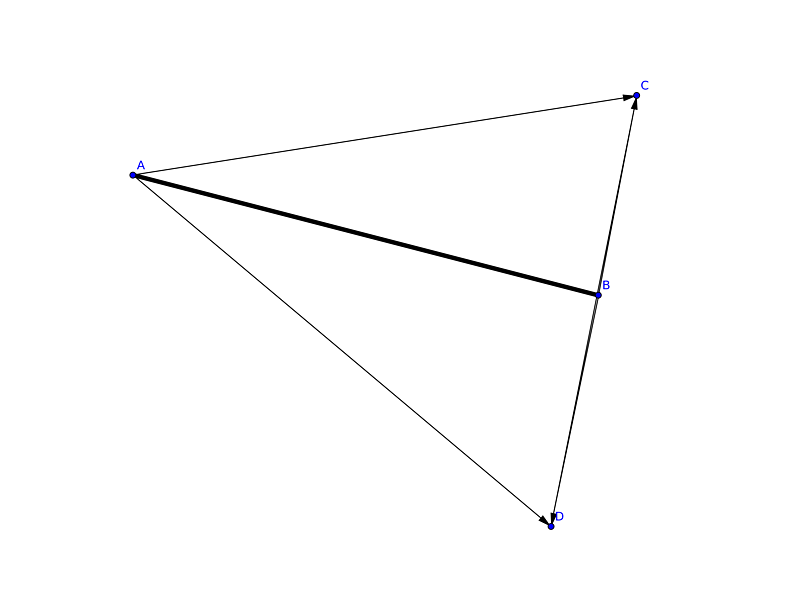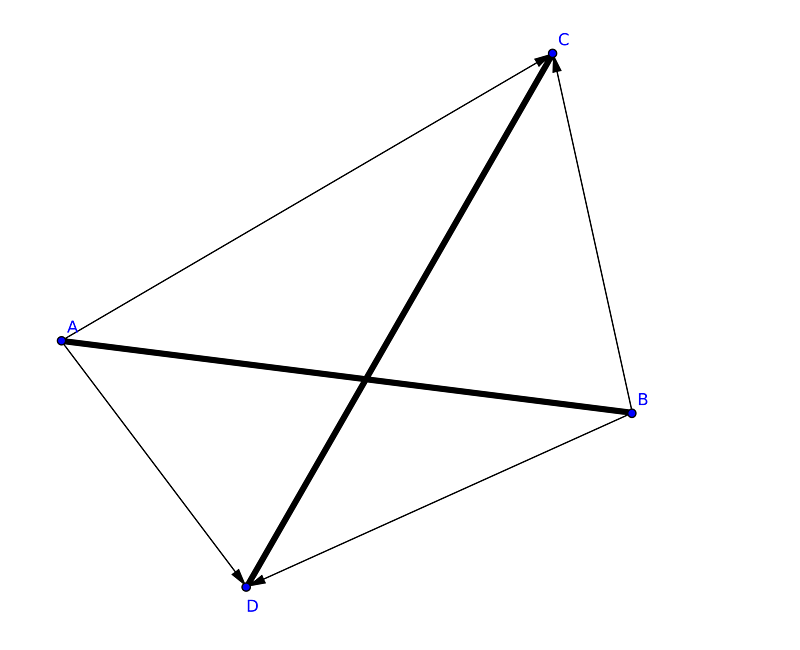*解析几何的巅峰

——(出处在最下方参考资料中)

## 题目：

###题目描述

###输入

###输出

###样例输入
3
1 5 2 9
1 3 2 4
5 6 7 8
5 7 7 7
2 5 1 0
9 4 2 9

###样例输出
disjointdisjoint
intersectintersect
disjoint

## 思路## 代码实现

#include <iostream>
#include <algorithm>
using namespace std;

//typedef pair<int,int> P

struct node
{
int x, y;

node() :x(0), y(0) {    };

friend istream& operator >> (istream& in, node& a);
};

istream& operator >> (istream& in, node& a)//重载流输入输出
{
while (!(in >> a.x >> a.y))
return in;

return in;
}

inline bool first_judge(node a, node b, node c, node d)
{//判断两个线段的投影，投影相交才有可能有交点

if (max(a.x, b.x) < min(c.x, d.x) || min(a.x, b.x) > max(c.x, d.x))
return false;

if (max(a.y, b.y) < min(c.y, d.y) || min(a.y, b.y) > max(c.y, d.y))
return false;

return true;
}

inline int mul_cross(node a, node b, node c)
{//计算向量ab, 向量ac 的叉乘
return (b.x - a.x)*(c.y - a.y) - (b.y - a.y)*(c.x - a.x);
}

inline bool IsCross(node a, node b, node c, node d)
{//判断是否有交点
if (!first_judge(a, b, c, d))
return false;

int tmp1 = mul_cross(a, c, d);
int tmp2 = mul_cross(b, c, d);

return tmp1 * tmp2 <= 0 ? true : false;
}

int main()
{
int t;
cin >> t;

while (t--)
{
node a, b, c, d;

cin >> a >> b >> c >> d;

IsCross(a, b, c, d) ? cout << "intersect" << endl : cout << "disjoint" << endl;
}

return 0;
}
Last modification：April 3rd, 2019 at 07:39 pm
If you think my article is useful to you, please feel free to appreciate